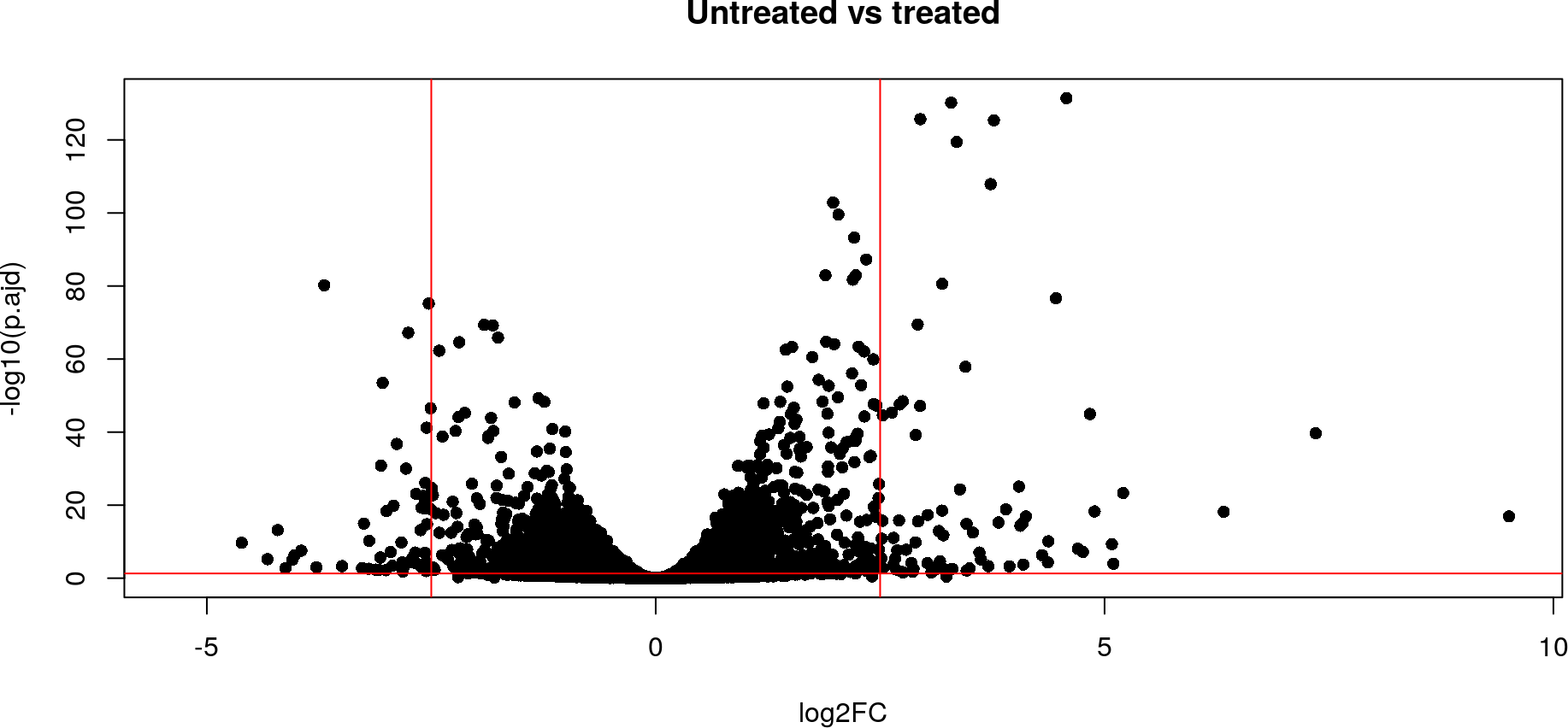# 1 Introduction

In this vignette we assume that the reader is already familiarized with the introduction vignette, but wants to know how can it help to answer other questions in other situations

We follow the same convention about names of the objects used `genesReact` and `genesKegg` are the list with information about the pathways the genes are involved in.

# 2 Merging similarities

If one calculates similarities with KEGG data and Reactome or other input for the same genes or clusters BioCor provides a couple of functions to merge them.

We can set a weight to each similarity input with `weighted.sum`, multiply them also using a weight for each similarity (with `weighted.prod`), doing the mean or just adding them up. Similarities allow us to apply a function to combine the matrices of a list. Here we use some of the genes used in the first vignette:

``````kegg <- mgeneSim(c("672", "675", "10"), genesKegg)
react <- mgeneSim(c("672", "675", "10"), genesReact)
## We can sum it adding a weight to each origin
weighted.sum(c(kegg["672", "675"], react["672", "675"]), w = c(0.3, 0.7))
##  0.7247289

## Or if we want to perform for all the matrix
## A list of matrices to merge
sim <- list("kegg" = kegg, "react" = react)
similarities(sim, weighted.sum, w = c(0.3, 0.7))
##            672        675         10
## 672 1.00000000 0.72472885 0.09976175
## 675 0.72472885 1.00000000 0.05258438
## 10  0.09976175 0.05258438 1.00000000
similarities(sim, weighted.prod, w = c(0.3, 0.7))
##           672          675           10
## 672 0.2100000 0.0173101952 0.0000000000
## 675 0.0173102 0.2100000000 0.0001239029
## 10  0.0000000 0.0001239029 0.2100000000
similarities(sim, prod)
##           672         675          10
## 672 1.0000000 0.082429501 0.000000000
## 675 0.0824295 1.000000000 0.000590014
## 10  0.0000000 0.000590014 1.000000000
similarities(sim, mean)
##            672        675         10
## 672 1.00000000 0.54121475 0.07125839
## 675 0.54121475 1.00000000 0.03991506
## 10  0.07125839 0.03991506 1.00000000``````

This functions are similar to `weighted.mean`, except that first the multiplication by the weights is done and then the `NA`s are removed:

``````weighted.mean(c(1, NA), w = c(0.5, 0.5), na.rm = TRUE)
##  1
weighted.mean(c(1, 0.5, NA), w = c(0.5, 0.25, 0.25), na.rm = TRUE)
##  0.8333333
weighted.sum(c(1, NA), w = c(0.5, 0.5))
##  0.5
weighted.sum(c(1, 0.5, NA), w = c(0.5, 0.25, 0.25))
##  0.625
weighted.prod(c(1, NA), w = c(0.5, 0.5))
##  0.5
weighted.prod(c(1, 0.5, NA), w = c(0.5, 0.25, 0.25))
##  0.0625``````

# 3 Assessing a differential study

In this example we will use the functional similarities in a classical differential study.

## 3.1 Obtaining data

We start using data from the RNAseq workflow and following the analysis comparing treated and untreated:

``````suppressPackageStartupMessages(library("airway"))
data("airway")
library("DESeq2")

dds <- DESeqDataSet(airway, design = ~ cell + dex)
dds\$dex <- relevel(dds\$dex, "untrt")
dds <- DESeq(dds)
## estimating size factors
## estimating dispersions
## gene-wise dispersion estimates
## mean-dispersion relationship
## final dispersion estimates
## fitting model and testing
res <- results(dds, alpha = 0.05)
summary(res)
##
## out of 33469 with nonzero total read count
## LFC > 0 (up)       : 2211, 6.6%
## LFC < 0 (down)     : 1817, 5.4%
## outliers        : 0, 0%
## low counts      : 16676, 50%
## (mean count < 7)
##  see 'cooksCutoff' argument of ?results
##  see 'independentFiltering' argument of ?results
pch = 16, xlab = "log2FC",
ylab = "-log10(p.ajd)", main = "Untreated vs treated"
)
logFC <- 2.5
abline(v = c(-logFC, logFC), h = -log10(0.05), col = "red")``````Figure 1: Volcano plot
The airway data

As we can see here there are around 4000 genes differentially expressed genes, some of them differentially expressed above 2^2.5.

## 3.2 Selecting differentially expressed genes

Usually in such a study one selects genes above certain logFC or fold change threshold, here we use the absolute value of 2.5:

``````fc <- res[abs(res\$log2FoldChange) >= logFC & !is.na(res\$padj), ]
fc <- fc[fc\$padj < 0.05, ]
# Convert Ids (used later)
genes <- select(org.Hs.eg.db,
keys = rownames(res), keytype = "ENSEMBL",
column = c("ENTREZID", "SYMBOL")
)
## 'select()' returned 1:many mapping between keys and columns
genesFC <- genes[genes\$ENSEMBL %in% rownames(fc), ]
genesFC <- genesFC[!is.na(genesFC\$ENTREZID), ]
genesSim <- genesFC\$ENTREZID
names(genesSim) <- genesFC\$SYMBOL
genesSim <- genesSim[!duplicated(genesSim)]
# Calculate the functional similarity
sim <- mgeneSim(genes = genesSim, info = genesReact, method = "BMA")
## Warning in mgeneSim(genes = genesSim, info = genesReact, method = "BMA"): Some
## genes are not in the list provided.``````

Once the similarities for the selected genes are calculated we can now visualize the effect of each method:

``````nas <- apply(sim, 1, function(x) {
all(is.na(x))
})
sim <- sim[!nas, !nas]

MDSs <- cmdscale(1 - sim)
plot(MDSs, type = "n", main = "BMA similarity", xlab = "MDS1", ylab = "MDS2")
up <- genes[genes\$ENSEMBL %in% rownames(fc)[fc\$log2FoldChange >= logFC], "SYMBOL"]
text(MDSs, labels = rownames(MDSs), col = ifelse(rownames(MDSs) %in% up, "black", "red"))
abline(h = 0, v = 0)
legend("top", legend = c("Up-regulated", "Down-regulated"), fill = c("black", "red"))``````